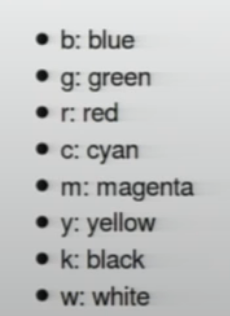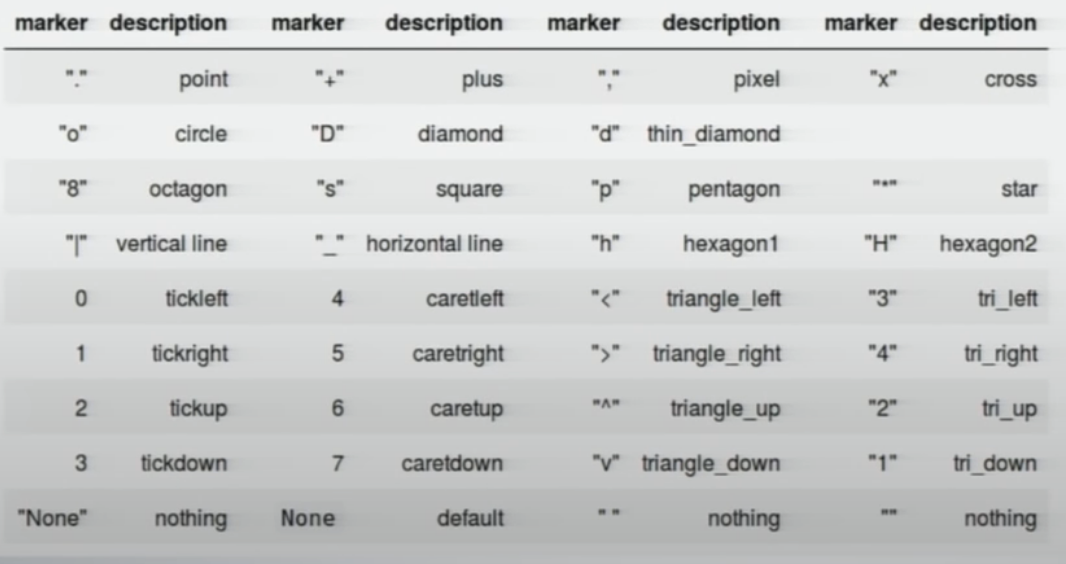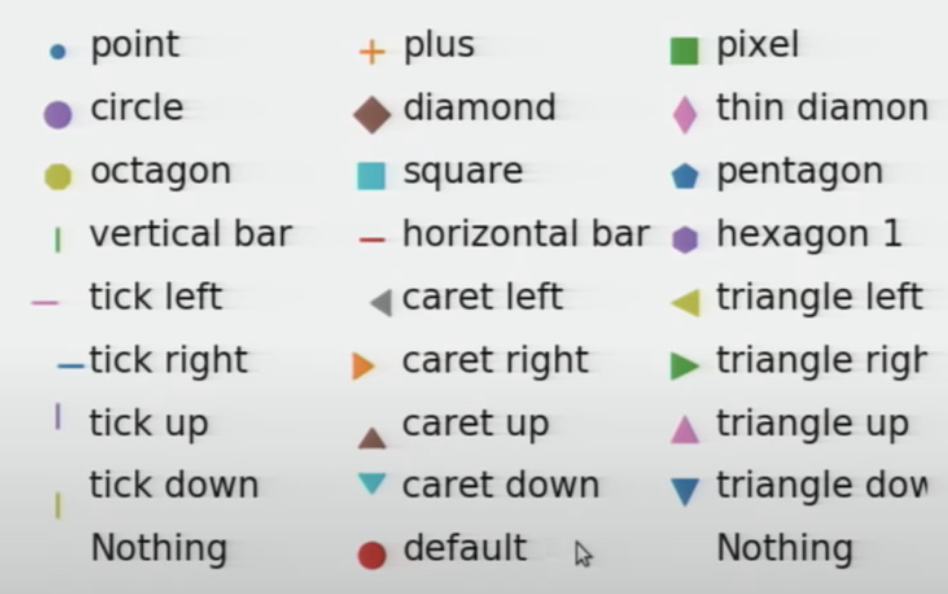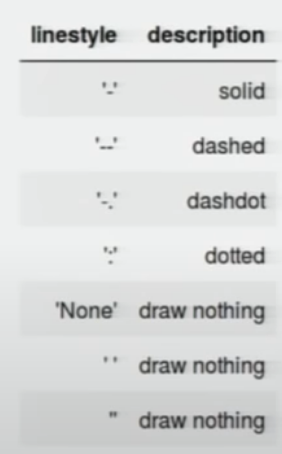<< Back to posts

# Matplotlib Tips + Tricks

## Lesser-known matplotlib tricks for frequent users of the Python library

Posted on August 13, 2022 • Tags:

These are all taken from this great 3-hr YouTube tutorial by Ben Root from SciPy 2018. I’ve condensed the main takeaways of the talk into the following list of key concepts / tricks that I hadn’t previously been aware of.

# General

To set figure aspect ratio to 2:1:

fig, ax = plt.subplots(figsize=plt.figaspect(0.5))


To loop through axes without axes notation:

fig, axes = plt.subplots(nrows=2, ncols=2)
for ax in axes.flat:
ax.plot(x,y)


To change z-order of plot (higher value = on top):

ax.plot(x, y, zorder=1)
ax.fill_between(x, y1, y2, zorder=2)


To get aes() ggplot-like dataframe processing:

df = {
'x' : ,
'y' : ,
}
ax.plot('x', 'y', data=df)


To modify the aesthetics of any “artist” (i.e. visual element on a plot) after creation:

bars = ax.bar(x, y, color='k', align='center')
for b, y in zip(bars, y):
if y < 0:
bar.set(color='blue')


To plot a bunch of points with the same marker style (regardless of whether you show a line or not), use plot(). Otherwise, use scatter()

To get LaTex, use an r string with $ for math: plt.title(r'$\sigma_i=1$')  To add/remove padding to plot: ax.margins(x=0.1,y=0)  To get x-data to take up same number of pixels as equivalent range in y-axis: ax.axis('equal')  To get chart element to not appear in legend, use the _nolegend_ label: ax.plot(x, y, label="_nolegend_")  To have two plots share axes: fig, axes = plt.subplots(1,2, sharex=True, sharey=True)  To have one plot have two different y-axis scales (“twinning”): fig, ax1 = plt.subplots() ax2 = ax1.twinx() ax1.plot(x,y) ax2.plot(x2,y2)  # Artists An artist is anything that is visible on the plot. There are two types of artists: 1. Primitives – Shapes • e.g. Rectangle, Polygon, Circle, Line 2. Containers – They are given a list of primitives to draw • e.g. Figure, Axes, Collection To get a list of properties for an artist$X\$:

plt.getp(X)


# Limits

Always set limits after you plot your data

# Ticks

• Tick (ticks) = location of tick label
• Tick Line (ticklines) = line that denotes location of the Tick
• Tick Label (ticklabels) = text dislabed at Tick
• Ticker = automatically determines Ticks + formats Tick Labels

# Colors## Colormaps

Colorbars must be added to fig, not ax b/c it will occur outside of the ax and “steal” room from it. By default, it steals space from the last ax

im = ax.imshow(data)
fig.colorbar(im)


To use bottom-left to be origin of image (rather than top-left):

im = ax.imshow(data, origin='lower')
fig.colorbar(im)


To get to be centered at 0 (i.e. map white to 0 for the red/blue seismic colormap), use vim, vmax to specify the range of the colormap

X = Some constant
im = ax.imshow(data, cmap='seismic', vmin=-X, vmax=X)


To get an array from a colormap:

from matplotlib import cm

# To get 'Blues' divided into 10 equally spaced shades, using full spectrum
colors = cm.get_cmap('Blues', 10)(np.linspace(0, 1, 10))


# Markers + Lines